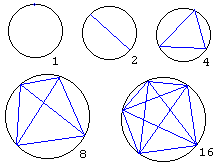## Partitioning a Circle

### ProblemN points are selected on a circle and connected by chords in all possible ways. The points are in a general position, in the sense that no three of those chords are concurrent, i.e., no three of them pass through the same point. Into how many region the chords partition the circle?

Let's denote the number of regions in a partition with N points R(N). As a quick inspection of a few small point sets shows R(1) = 1, R(2) = 2, R(3) = 4, R(4) = 8, R(5) = 16. The pattern appears to emerge: R(N) = 2N-1. However, R(6) = 31. An attempt at inductive reasoning, i.e., an attempt to generalize from a finite sample of verified examples proves to be misleading.

One solution to this problem links it to partitioning of convex polygons. Here I'll give a solution that depends on Euler's formula. We've proven that

F - E + V = 2

for a polyhedron with F faces, E edges and V vertices. Considering a polygon as a projection of a polyhedron with just one face hidden, the Euler's formula for polygons becomes:

F - E + V = 1

This is the one we shall be referring to below. The formula remains valid if edges allowed to be curved so that it applies to general planar graphs.

I'll use the standard notation C(n, r) for the number of all possible unordered selections of r elements out of a set of n elements. These are called r-combinations. C(n, r)'s appear as coefficients in the Binomial Theorem:

C(n, r) =
 n!r!(n - r)!

As usual, k! = 1·2·3·...·k is the k factorial.

For N points, there are exactly C(N, 4) intersections of chords inside the circle, since each set of 4 points gives rise to just one such intersection. Then the total number of vertices in the figure V = C(N, 4) + N. To count the number E of edges, we count their ends. Four edges meet at each of the internal C(N, 4) vertices and (N + 1) meet at the points on the circle. Since each edge has two ends, this gives 2E = 4C(N, 4) + N(N + 1). Using the Euler's formula:

F = E + 1 - V = (4C(N, 4) + N(N + 1))/2 + 1 - (C(N, 4) + N).

which simplifies to C(N, 4) + C(N, 2) + 1.

### References

1. R. K. Guy, A Strong Law of Small Numbers, in The Lighter Side of Mathematics, R.K.Guy and R.E.Woodrow, eds, MAA, 1994• Counting: The Beginning of Mathematics
• Children's Counting Blocks Are Available
• The Idea of Counting
• Tribute to Invariance
• Dollars in Pockets
• Counting Triangles
• Counting Triangles II
• 100 Grasshoppers on a Triangular Board
•